##### Postgres Length Functions With Practical Examples

In PostgreSQL, you can perform various operations on the strings, such as concatenating various strings, finding the length of strings, extracting substrings from strings, etc. To manipulate the string data efficiently, Postgres offers a wide range of built-in string functions, such as CONCAT(), LENGTH(), SUBSTRING(), etc.

Several length functions will be explained by examples in this blog post.

What are Length Functions in PostgreSQL?

Postgres offers various built-in length functions to calculate the length of a string. The length functions, along with the description listed below:

- LENGTH(): The length function takes a string as an argument and retrieves the length of the input string(total number of characters, including white spaces).
- CHAR_LENGTH(): The CHAR_LENGTH() or CHARACTER_LENGTH() function calculates the string’s length in terms of characters(same as the LENGTH() function).
- BIT_LENGTH(): The BIT_LENGTH() function retrieves the length of the string in terms of bits.
- OCTET_LENGTH(): The OCTET_LENGTH() function accepts a string as an argument and retrieves the string’s length in terms of bytes.

Example 1: How Do I Use the LENGTH() Function in Postgres?

Let’s comprehend the working of the Postgres LENGTH() function by passing a string as an argument to it:

`SELECT LENGTH('Hello! Welcome to commandprompt.com');`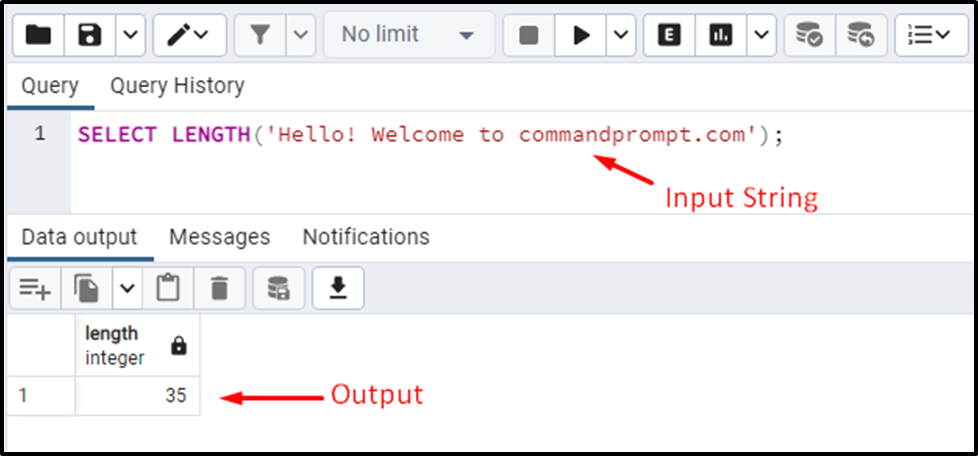The snippet provided above shows that the LENGTH() function retrieves the string’s length by counting the number of characters, including spaces.

Example 2: How Do I Use the CHAR_LENGTH() Function in Postgres?

The CHAR_LENGTH() function works the same as the LENGTH() function, i.e., it accepts a string as an argument and retrieves the length of the string by counting the total number of characters:

`SELECT CHAR_LENGTH();`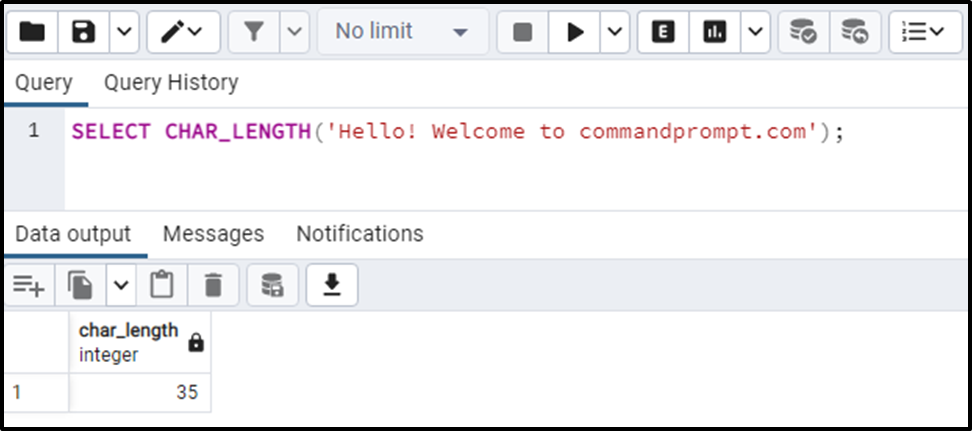The output authenticates the working of the CHAR_LENGTH() function as it retrieves the total numbers present in the input string.

Example 3: How Do I Use the BIT_LENGTH() Function in Postgres?

Let’s pass a string to the BIT_LENGTH() function and see how it works in Postgres:

`SELECT BIT_LENGTH('Hello! Welcome to commandprompt.com');`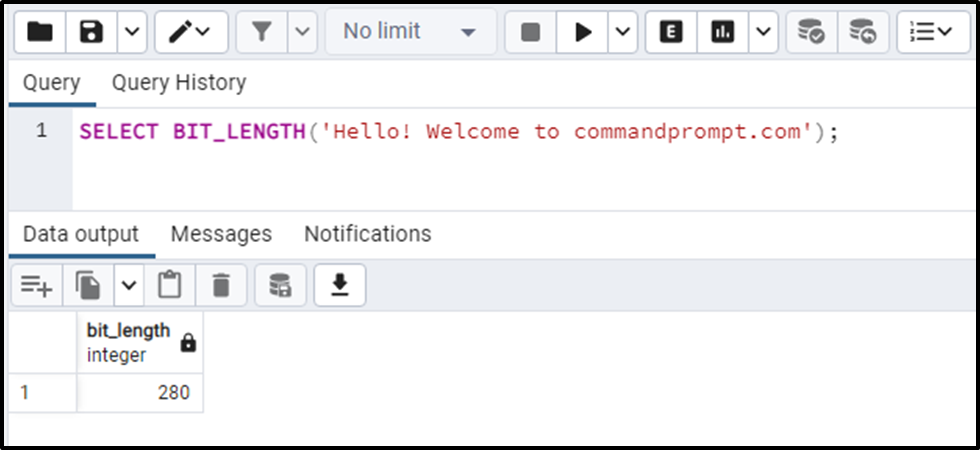The output shows that the BIT_LENGTH() function retrieves the string length in bits.

Example 4: How Do I Use the OCTET_LENGTH() Function in Postgres?

Let’s pass the same string to the OCTET_LENGTH() and see how it works:

`SELECT OCTET_LENGTH('Hello! Welcome to commandprompt.com');`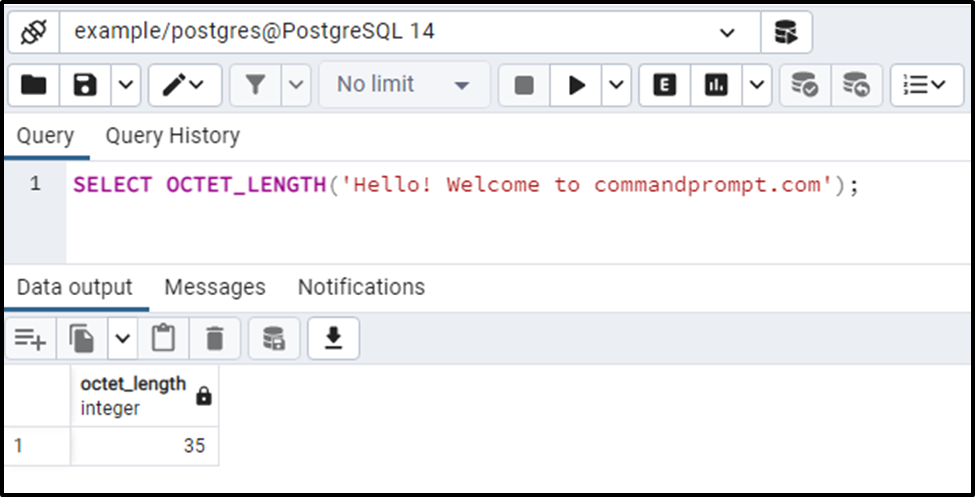The OCTET_LENGTH() function retrieves the output in bytes.

To comprehend the working of each function, let’s consider the below example.

Example 5: How Do I Use the OCTET_LENGTH() Function in Postgres?

Firstly, let’s create a table named “sample_tab” with two columns: id and value:

```CREATE TABLE sample_tab(
id INT,
value VARCHAR);```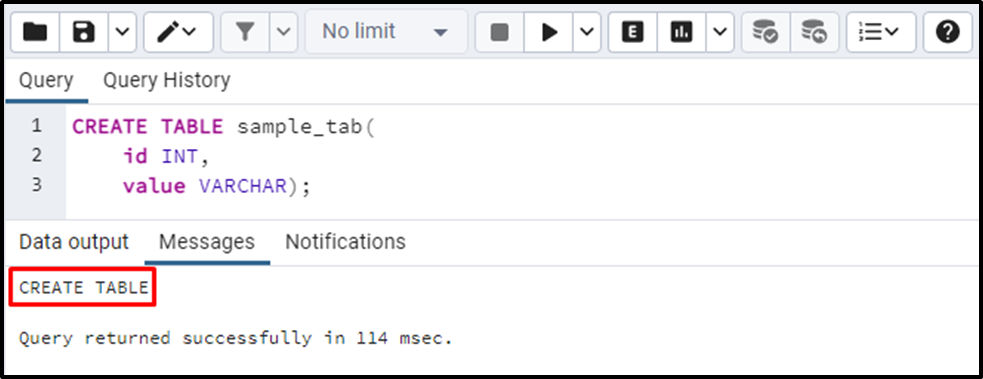Let’s insert a bulk of values into the “smaple_tab” table:

```INSERT INTO sample_tab (id, value)
VALUES (1, ‘a’),
(2, ‘₩'),
(3, ‘hello world’);```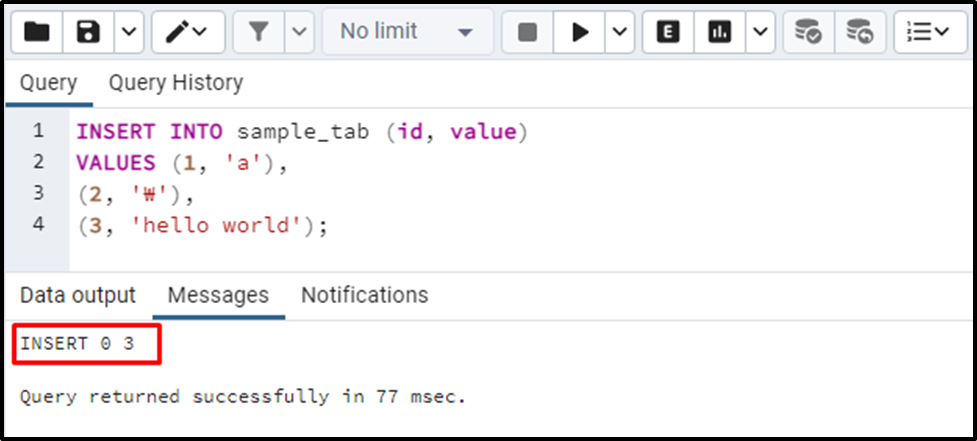Three values have been inserted into the “smaple_tab”. Now let’s implement each of the length functions on the table’s data to clarify their working:

```SELECT value, LENGTH(value),
CHAR_LENGTH(value),
BIT_LENGTH(value),
OCTET_LENGTH(value)
FROM sample_tab;```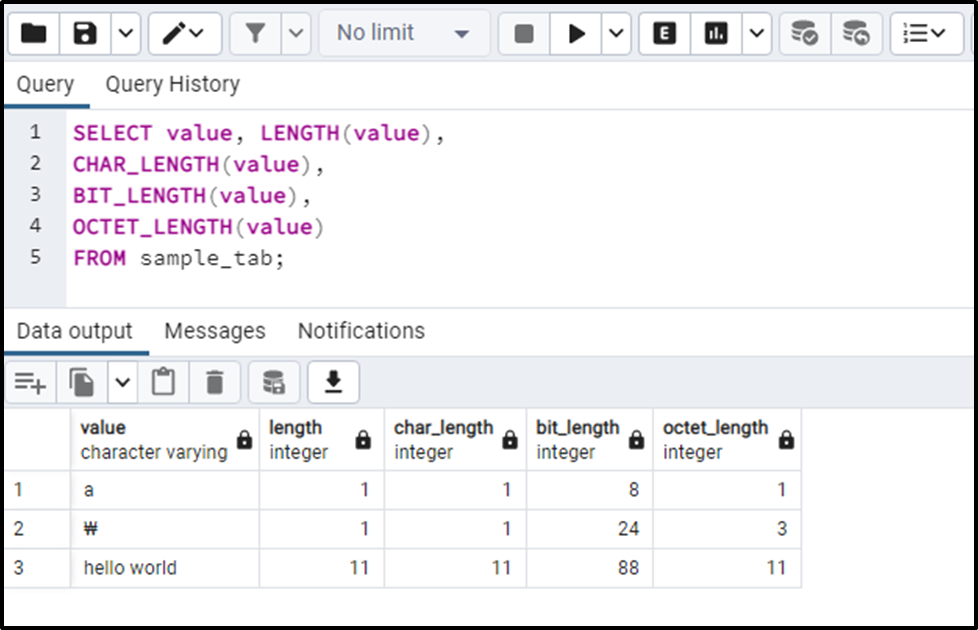“₩” is a special symbol that takes three bytes:

- So, when it is passed to LENGTH() and CHAR_LENGTH() functions, they consider it a single character and hence retrieve ‘1’ as the total length.
- When the “₩” symbol is passed to the BIT_LENGTH() function, it calculates and retrieves the total number of bits as output. Since one byte is equal to eight bits, therefore, it retrieves 24 as output (3*8).
- The OCTET_BYTE() retrieves “3” since the “₩” symbol takes three bytes.

Conclusion

Postgres offers various built-in length functions to calculate the length of a string, such as LENGTH(), BIT_LENGTH(), CHAR_LENGTH(), and BYTE_LENGTH(). The LENGTH() and CHAR_LENGTH() functions take a string as an argument and retrieve the length of the input string(total number of characters, including white spaces). The BIT_LENGTH() function retrieves the length of the string in terms of bits. While the OCTET_LENGTH() function accepts a string as an argument and retrieves the string’s length in terms of bytes. This write-up demonstrated the working of various length functions using practical examples.